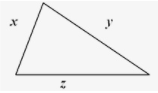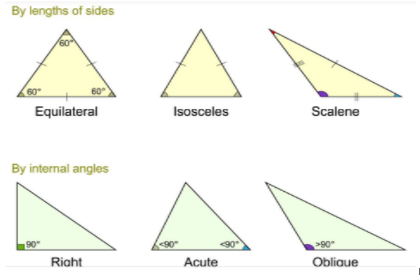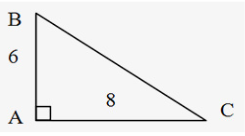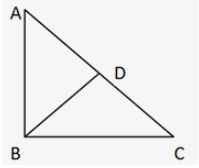• Home
• Triangle Based Ques...

# Triangle Based Questions For Aptitude Exams for DU JAT and IPM

Author : Palak Khanna

Updated On : August 1, 2023

SHARE

# Triangle based questions for DUJAT and IPM

Entrance exams give a reasonably good amount of weightage to quantitative aptitude.  In the IPMAT exam, 40 questions are being asked in the MCQ form and 20 questions in short answer type from the quant section. In the DUJAT exam, quantitative ability comprises a total of  25 questions for 100 marks. Therefore, preparing well for topics under quantitative ability becomes important for securing good marks.
Geometry is one of the important sections in the quant section from which questions are asked  every year. Questions from Lines, Angles and Triangles are asked in Geometry. This article provides a blueprint about how to go on preparing triangle based questions, properties of triangles, explained answers, etc.
Triangle is a sub-topic under Geometry with a very wide domain. Before attempting questions on Triangle, your basics of the properties of triangle should be crystal clear.

## Introduction

A triangle is a plane figure bounded by three lines and joins three non-collinear points. Triangles come in a variety of shapes and sizes, each with its own set of characteristics. Some features, however, apply to all triangles. One such feature is that the total of any two triangle sides is always bigger than the triangle's third side. It is depicted as follows:
Here,  x + y > z, x + z > y, and y + z > x.## Types of Triangles:

Triangles are divided into three sorts based on their sides:

• Scalene Triangle: A scalene triangle is a triangle in which none of the three sides are equal.
• Isosceles Triangle: An isosceles triangle is a triangle with in which two sides are equal sides.

The angles opposite the congruent sides are congruent in an isosceles triangle. In addition, if two triangle angles are identical, the sides opposite them are likewise equal.

• Equilateral Triangle: An equilateral triangle is one in which all of the angles and sides are the same. If AB = BC = AC in ABC. Then ∠ABC = ∠BCA = ∠CAB = 60 degrees.

See the images given below:## Triangles are classified into three categories based on their angles:

• Acute Triangle: When the three angles of a triangle are acute, that is, less than 90 degrees, the triangle is called an acute triangle.
• Obtuse Triangle: When one of a triangle's three angles is obtuse (larger than 90°), the triangle is said to be an obtuse angled triangle. An obtuse angled triangle's remaining two angles are always acute.
• Right Triangle: A right angled triangle is one in which one of the three angles is a right angle (i.e. exactly 90°). The right angled triangle's remaining two angles are always acute angles. Pythagorean Theorem is an important characteristic of right-angled triangles. It claims that the sum of the squares of the Base and Perpendicular in a right-angled triangle equals the square of the triangle's Hypotenuse.In the above right angled triangle, (AB)2 + (AC) 2 = (BC)2.

Example:  if AB = 6 and AC= 8, then BC= 10, because 62 + 82 = 36 + 64 = 100 = (BC) 2

There are some Pythagorean triplets in the questions that are regularly used. It is preferable to learn these triplets by heart.

3, 4 & 5
5, 12 & 13
7, 24 & 25
8, 15 & 17
9, 40 & 41
11, 60 & 61
12, 35 & 37
16, 63 & 65
20, 21 & 29
28, 45 & 53.

Any multiple of these Pythagorean triplets will also be a Pythagorean triplet, i.e. if we say 5,12,13 is a triplet, we may multiply all of these numbers by 4 and get 20, 48, 52 which is also a Pythagorean triplet.

Special features of Right Angled Triangle

### Triangle 45-45-90

• ·Two of the angles are 45 degrees, while the third is a right angle.
• ·This triangle's sides will be in the ratio 1: 1: 2 accordingly.
• ·Because two angles are equal, this is also known as an isosceles right-angled triangle.

### Triangle of 30-60-90 degrees

• ·This is a right-angled triangle since one angle equals 90 degrees.
• ·This triangle's angles are in the ratio 1: 2: 3, and the sides opposite these angles are in    the ratio 1: 3: 2, correspondingly.
• ·Because all three angles are distinct, this is a scalene right-angled triangle.

## Important properties of triangles

• The sum of the 3  interior angles of a triangle equals 180°.  Any triangle's total exterior angles are equal to 360°.

In ∆ABC, ∠ABC + ∠BAC + ∠ACB = 180°

•  Sum of an inner angle and the adjacent outside angle equals 180 degree.
•  When two external angles have the same vertex, they are said to be congruent.
• The sum of the measures of the two interior angles (called remote interior angles) of the triangle that are not contiguous to it equals the measure of an exterior angle.
• In a triangle, the total of any two sides is always greater than the third side.
•  The difference between any two sides is always less than the difference between the third and fourth sides.
• The sum of a triangle's two inner opposed angles equals the triangle's outer angle.
• The shortest side is the side opposite the smallest interior angle, and vice versa. Similarly, the longest side is the side opposite the biggest interior angle, and vice versa. This side is known as the hypotenuse in a right-angled triangle.
• Ratios of sides = ratios of heights = ratios of medians = ratios of angle bisectors = ratios of inradii = ratios of circum radii; when two triangles are similar to each other.
• The two triangles drawn from the vertex of the right angle to the largest side, the Hypotenuse, on each side of the perpendicular are comparable to each other and likewise to the larger triangle.∆ DBA is similar to ∆ DCB which in turn is similar to ∆ BCA.

The geometric mean of the segments into which the hypotenuse is divided is the altitude from the vertex of the right angle to the hypotenuse.
i.e. (DB)2 = AD * DC

Triangle congruence:  Two triangles are said to be congruent if and only if the sides and angles of the first triangle are equal to the corresponding sides and angles of the other triangle.

• ·A triangle's two sides and included angle are equivalent to the two sides and included angle of another triangle (SAS).
• ·Two angles and one side of a triangle are equal to two angles and the corresponding side of the other triangle, respectively (AAS).
• ·The three sides of one triangle are congruent with the three sides of the other triangle (SSS).
• ·The hypotenuse and one side of a right triangle are congruent to the hypotenuse and one side of another right triangle (RHS).

Triangle similarity: Two triangles are considered to be similar if they are similar in shape. These triangles' corresponding angles are equal, but their corresponding sides are merely proportionate.

Congruent triangles are all similar, however not all similar triangles are congruent.

• · A triangle's three sides are proportional to the other triangle's three sides (SSS).
• ·A triangle's two angles are equal to the opposite triangle's two angles (AA), respectively.
• ·The included angles are equal and two sides of a triangle are proportional to two sides of another triangle (SAS).
• Circumcenter :  The circumcenter of a triangle is the center of the circumcircle. The intersection of the perpendicular bisectors can be used to find it.
• Incenter : The incenter of a polygon is a point that represents the center of the incircle. The inradius of the incircle is the corresponding radius of the incircle

## Some important formulas

• When all sides are known : Heron’s formula = {s (s - a) (s - b) (s - c)}1/2  where, s = (a+b+c)/2
• When two sides and corresponding angle is known = ½ a*c*sinθ
• When all medians are known = 4/3 * {s(s – m1) (s – m2) (s –m3)}1/2

Note: Where, s = (m1+m2+m3)/2 ;  m1,m2,m3 are three median of the Triangle.

• Area of a Equilateral Triangle = Root of (3) / 4 * (Side)2
• Perimeter of an Equilateral Triangle = 3 * Side
• Area of an Isosceles Triangle = b/4 * root of 4a2 – b2
• Area of Triangle = 1/2 * Base * Height

## Tips and Recommended Study material

Do not try to attempt all the questions as the cut off is never 100%. Smartly pickup the low hanging fruits. Attempt questions in which you are confident first. Rather than attempting all the questions, focus on the accuracy of the questions which you attempt.

Here is the list of books recommended by toppers-

• Crack the IIM Indore - IPM Entrance Exam.
• NCERT class XI and XII
• RD Sharma
• RS Aggarwal
• ML Khanna

You can learn some of the super tricks to solve Triangle-based questions for DUJAT and IPM on the youtube channel of Supergrads. Click here to brush up your concepts and solve super questions based on Triangles.
Some relevant questions with explanation

1. The area of a triangle is 615 cm2. Find length of the perpendicular that is dropped on that particular side from the opposite vertex if one of its sides is 123 centimetres?
A. 10 cm                       B. 50 cm                       C. 0.16 cm                     D. 0.2 cm
Area = 615cm2.   Base = 123 cm  Perpendicular = ?
Also A = 1/2 * B * H  ∴ 615 = 1/2 * 123 * H   ⇒ H = 10 cm.

2. Two sides of an isosceles triangle equal 12.5 cm each and the third side is 20 cm. Find the area of the triangle?
A. 25 cm2                B. 75 cm2                  C. 12 cm2                                  D. 10 cm2
Answer : Lengths of the sides are 12.5 cm, 12.5 cm and 20 cm. ∴ s = (12.5 + 12.5 + 20) / 2 ⇒ s = 22.5. Area = 75 cm2

3. One of the sides of a triangle is 3 cm and the perimeter is 8 cm. If the area of the triangle is maximum then the other two sides are:
A.     (3,3)                      B. (7,5)                         C.(2/3,2)                              D.(5/2,5/2)
Some questions of geometry borrow concepts from Algebra.
Let a, b and c be three sides of the triangle. Perimeter= 8 cm, so a+b+c = 8 cm, a+b+3 = 8 cm, a+b= 5 cm. If we know the angle between two sides and the angle between two sides, then we can use the formula A= ½ ab sin θ. Now the angle between two sides is constant, we cannot change that. But when product of a and b is maximum then area will be maximum. Product of a and b is maximum when a=b. Now we know that a+b = 5. This implies that a=b= 5/2 .
Option D. (5/2,5/2) is the answer.

4. There are obtuse angle triangles with sides as 8 cm, 15 cm, and the third side is unknown. If the length of the third side is an integer, how many such triangles exist?
A.     20
B.      10
C.     13
D.     15

To solve this question, one should know the general properties of triangles and specific properties of obtuse angled triangles. Two properties of obtuse angled triangle will be used here:

• c² > a² + b²  , where c is the longest side; and
• Sum of any two sides is greater than third side : a + b > c, b + c > a , a + c >b

Let the third side be x cm. Either 15 cm will be the largest side of x. Let's consider one by one
Let x be the largest side : 8 + 15 > x cm  or 23 > x cm or x< 23 cm. Because x is an integer, it can be at most 22. x2 > 8*8 + 15*15   x2 > 64 + 225   x2  > 289  x > 17 . Now we have 17 < x < 23. So, 18, 19, 20, 21 and 22 are the 5 possible values.

Let the largest side be 15 cm. 8 + x > 15 cm or x > 7 cm. c² > a² + b² => 15*15 > 8*8 + x*x => 225 > 64 + x2   or x2  < 161 or x < 12.56 cm . So,  7 cm < x < 12.56 cm; so,  8,9,10,11,12 are possible values of x.
Total number of possible values = 5 + 5 = 10. Option B is the right answer.

5. A circle is put inside an equilateral triangle with side 24 cm, touching its sides. Find the area of the remaining portion of the triangle?
A.   144√3 - 48π cm2
B.   122√3 - 36π cm2
C.  147√3 - 36π cm2
D.  130√3 - 47π cm2

The radius of the circle is one third of the height of the triangle, when it is inscribed in an equilateral triangle.
Height of an equilateral triangle(h) = √3/2 * a, (a is the length of  side of triangle)
h = √3/2 * 24 = 12 √3.

Radius of Circle (r) = 1/3 * h = 12 √3/3 = 4 √3.
Area of an equilateral triangle (A) = √3/4 * a2, (a is the length of a side of triangle)
A = √3/4 * (24)2 = √3 * 144.
Area of the Circle (a) = π r2., ( r is the radius of the circle)
a = π (4 √3)2 = 48 π.

Area of rest of the portion of the triangle= A – a = √3 * 144 - 48 π = 144 √3 - 48 π. Option A is the correct answer.